Courses

# Test: Fluid Mechanics Level - 4

## 25 Questions MCQ Test Civil Engineering SSC JE (Technical) | Test: Fluid Mechanics Level - 4

Description
This mock test of Test: Fluid Mechanics Level - 4 for Civil Engineering (CE) helps you for every Civil Engineering (CE) entrance exam. This contains 25 Multiple Choice Questions for Civil Engineering (CE) Test: Fluid Mechanics Level - 4 (mcq) to study with solutions a complete question bank. The solved questions answers in this Test: Fluid Mechanics Level - 4 quiz give you a good mix of easy questions and tough questions. Civil Engineering (CE) students definitely take this Test: Fluid Mechanics Level - 4 exercise for a better result in the exam. You can find other Test: Fluid Mechanics Level - 4 extra questions, long questions & short questions for Civil Engineering (CE) on EduRev as well by searching above.
QUESTION: 1

Solution:
QUESTION: 2

Solution:
QUESTION: 3

### A large metacentric height in a vessel

Solution:
QUESTION: 4

The time of oscillation of a floating body is given by
Where
K = Radius of Gyration of the floating body about its centre of gravity
h = Metacentric height of the floating body

Solution:
QUESTION: 5

A cylindrical body of cross-sectional area A height H and density ρs is immersed to a depth h in a liquid of density ρ and tied down to bottom with a string then the tension in the string is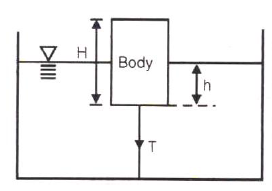Solution:
QUESTION: 6

There is no geometrical distinction between the streamline, pathline and streakline in case of

Solution:
QUESTION: 7

Stream line, path line and streak line are identical when the

Solution:
QUESTION: 8

Match List I with List II and select the correct answer using the codes given below the lists: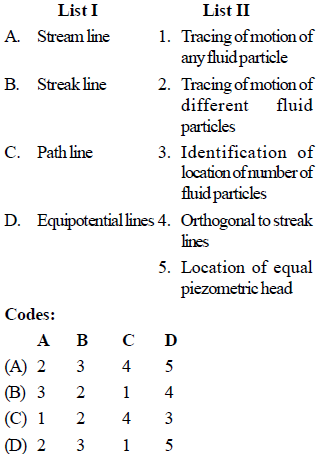Solution:
QUESTION: 9

The continuity equation in differential form is

Solution:
QUESTION: 10

Under which of the following conditions will the equation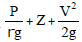= constant, will be valid in the whole flow field ?

1. Flow is rotational

2. Flow is irrotational

3. Flow is incompressible

5. Flow is laminar

Solution:
QUESTION: 11

​Venturimeter consists of following parts :

1. Diverging part

2. Throat

3. Main inlet pipe

4. Short converging part

Select the correct sequence of parts:

Solution:
QUESTION: 12

The range for coefficient of discharge (Cd) for a venturimeter is

Solution:
QUESTION: 13

In the siphon in figure below assuming ideal flow, the pressure PBSolution:

As PA = PC both have atmospheric pressure and keeping other factors of energy constant, as liquid is flowing from B to C, PB<PC
Hence PB<PA

QUESTION: 14

The flow of fluid through a pipe is laminar when

Solution:
QUESTION: 15

An oil of kinematic viscosity 0.5 stokes flows through a pipe of 4 cm diameter. The flow is critical at a velocity of about

Solution:
QUESTION: 16

In the Navier-Stokes equations, the forces considered are

Solution:
QUESTION: 17

Navier-Stoke's equation represents the conservation of

Solution:

The Navier-Stokes equations represent the conservation of momentum, while the continuity equation represents the conservation of mass.

QUESTION: 18

For laminar flow between two fixed parallel plates, the flow velocity

Solution:

Explanation : Laminar flow, type of fluid (gas or liquid) flow in which the fluid travels smoothly or in regular paths, in contrast to turbulent flow, in which the fluid undergoes irregular fluctuations and mixing. ... The fluid in contact with the horizontal surface is stationary, but all the other layers slide over each other.

The velocity profile is a parabola.

QUESTION: 19

Capillary tube viscometers used for measurement of viscosity are based on

Solution:
QUESTION: 20

The kinematic viscosity of oil of specific gravity .8 is .0005 .This oil is used for lubrication of shaft of diameter .4 m and rotates at 190 rpm. Calculate the power lost in the bearing for a sleeve length of 90mm. The thickness of the oil film is 1.5mm.

Solution:

Power lost= torque * angular velocity
= shear stress * area* radius* angular velocity
Shear Stress = viscosity* velocity gradient
Power lost= 0.0005*0.8*1000* 2*3.142*190/60*0.2*3.142*0.23 * 190/60
= 477.65 Watts.

QUESTION: 21

Pressure drop in a 100 mm diameter horizontal pipe is 50 kPa over a length of 10 m. The shear stress at the pipe wall is

Solution: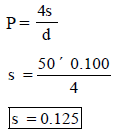QUESTION: 22

The pressure drop for a relatively low Reynolds number flow in a 600 mm, 30 m long pipe line is 70 kPa. What is the wall shear stress?

Solution:

Use,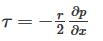QUESTION: 23

The body whose surface does not coincide with the streamline when places in a flow is called as

Solution:
QUESTION: 24

Shear velocity is

Solution:
QUESTION: 25

The head loss in a sudden expansion from 4 cm diameter pipe to 6 cm diameter pipe in terms of velocity V1 in the smaller diameter pipe is:

Solution: